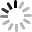# SVG D3.js - 繪製線段

## 使用 line() 畫線

``````var data = [
{x:10,y:10},
{x:50,y:100},
{x:60,y:50},
{x:100,y:30}
];
``````

``````  var svg = d3.select('body')
.append('svg')
.attr({
'width': 800,
'height': 800
});
``````

``````  var line = d3.svg.line()
.x(function(d) {
return d.x;
})
.y(function(d) {
return d.y;
});
``````

``````  svg.append('path')
.attr({
'd': line(data),
'y': 0,
'stroke': '#000',
'stroke-width': '5px',
'fill': 'none'
});
``````## .interpolate() 的模式

• linear：預設值，就是剛剛的演示。
• linear-closed：封閉的線段。
• step：一律以水平線段或垂直線段顯示，採中間點的方式做連接。
• step-before：以起始點垂直畫線方式開始。
• step-after：以終點垂直畫線方式結束。
• basis：基於 B-spline 的定義長出曲線。
• basis-open：開放的 B-spline，不包含起點與終點 ( 大於三個點 )。
• basis-closed：封閉的 B-spline。
• bundle：基於 B-spline 之上，但包含了 tension 參數。
• cardinal：基於 Cardinal spline 的線段。
• cardinal-open：開放的 Cardinal spline，不包含起點與終點 ( 大於三個點 )。
• cardinal-closed：封閉的 Cardinal spline。
• monotone：基於 cubic interpolation 的線段。

• linear-closed

``````  var line2 = d3.svg.line()
.x(function(d){
return d.x;
})
.y(function(d){
return d.y;
})
.interpolate('linear-closed');
``````• step ( 圖中紅色的點為 data 的座標提示，程式碼與上面相同，差別只在於 interpolate 的模式名稱 )：• step-before• step-after• basis• basis-open• basis-closed• bundle• bundle + tension

``````  var line2 = d3.svg.line()
.x(function(d){
return d.x;
})
.y(function(d){
return d.y;
})
.interpolate('linear-closed')
.tension(2);
``````• cardinal：• cardinal-open• cardinal-closed• monotone